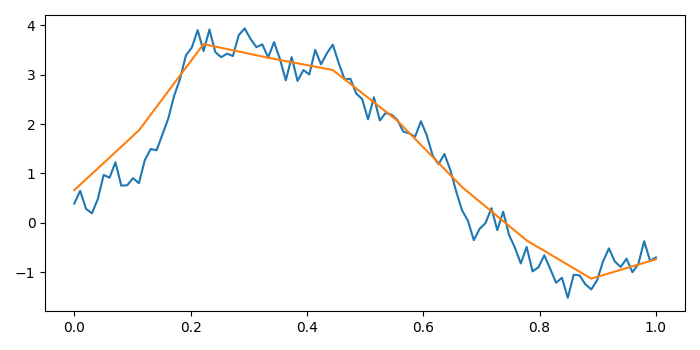# Plotting two different arrays of different lengths in matplotlib

MatplotlibPythonData Visualization

#### Python Data Science basics with Numpy, Pandas and Matplotlib

Most Popular

63 Lectures 6 hours

#### Data Visualization using MatPlotLib & Seaborn

11 Lectures 4 hours

#### MatPlotLib with Python

9 Lectures 2.5 hours

To plot two different arrays of different lengths in matplotlib, we can take the following steps −

• Set the figure size and adjust the padding between and around the subplots.
• Create y1, x1, y2 and x2 data points using numpy with different array lengths.
• Plot x1, y1 and x2, y2 data points using plot() method.
• To display the figure, use show() method.

## Example

import numpy as np
import matplotlib.pyplot as plt

plt.rcParams["figure.figsize"] = [7.00, 3.50]
plt.rcParams["figure.autolayout"] = True

y1 = (np.random.random(100) - 0.5).cumsum()
y2 = y1.reshape(-1, 10).mean(axis=1)

x1 = np.linspace(0, 1, 100)
x2 = np.linspace(0, 1, 10)

plt.plot(x1, y1)
plt.plot(x2, y2)

plt.show()

## Output

It will produce the following output